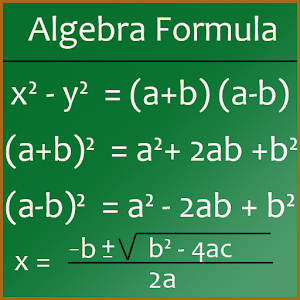# Maths Algebra Formula - Lumos Educational App Store4.11
Price -
\$

#### DESCRIPTION:

The app lists all the important Maths Algebra formulas. Its very useful for student to save valuable time. This App contains following formulas : 1) Arithmetic Progressoin 2) Arithmetic Operatopms 3) Law of Exponent 4) Factoring Formula 5) Absolute Value 6) Logarithms Properties 7) Complex Numbers 8) Radical Properties 9) Inequalities Properties 10) Quadratic Formula 11) Rules of Zeros

#### OVERVIEW:

Maths Algebra Formula is a free educational mobile app By simpleApps.It helps students in grades HS practice the following standards HSN.RN.A.1,HSN.RN.A.2,HSN.RN.B.3.

This page not only allows students and teachers download Maths Algebra Formula but also find engaging Sample Questions, Videos, Pins, Worksheets, Books related to the following topics.

1. HSN.RN.A.1 : Explain how the definition of the meaning of rational exponents follows from extending the properties of integer exponents to those values, allowing for a notation for radicals in terms of rational exponents. For example, we define 51/3 to be the cube root of 5 because we want (51/3)3 = 5(1/3)3 to hold, so (51/3)3 must equal 5.

2. HSN.RN.A.2 : Rewrite expressions involving radicals and rational exponents using the properties of exponents..

3. HSN.RN.B.3 : Explain why the sum or product of two rational numbers is rational; that the sum of a rational number and an irrational number is irrational; and that the product of a nonzero rational number and an irrational number is irrational.

HS

#### STANDARDS:

HSN.RN.A.1
HSN.RN.A.2
HSN.RN.B.3

Developer: simpleApps

Software Version: 1.3

Category: Education

### RELATED APPS